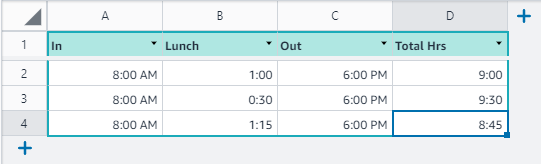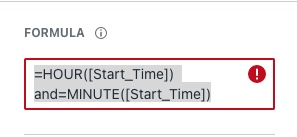# How to subtract one time from another time to get duration?

Hi Honeycoders,
I'm not sure if this has bneen asked before, I couldn't find any post about it.
I'm trying to build a timesheet app and have a start time and an end time. When I subtract one from the other I get very strange results

The sub total = [End] - [Start]-[lunch]
Both end and Start are Time
Lunch is number to 2 decimal places.
Sub Total is formatted as a number to 2 decimal places
When I try and get results , even from [End] - [Start] I get funny results.
In Google Sheets date/time or time is a single number and you have to use a conversion factor to get the correct result, I'm not sure how Honeycode manages this.
Any advice would be greatly appreciated.
Thank you in anticipation,

Hello @maxd-4943,

I believe the issue is in the Lunch variable type. It should be date as well but you can remove the AM / PM using column formats. please find the screenshot for more clarification.
All formats should be date, even the Total Hrs. Just change how to display the time format.Regards,
Meezal

Hi Meezal,
Thank you for your reply. I did go down that track BUT! Try putting in "0" lunch hours and it defaults to 12:00:00AM so that messes up the formula.
I ended up using =HOUR([END]) and =MINUTE([END]) to convert the End and Start to lowest common denominator of Minutes and then multiplied Lunch bu 60 to convert that to minutes, then after doing arithmatic dividing the Total hours by 60 to bring it to Fractional hours, that worked.
kind regards,
Max

3 Likes

Hello Max,

Can you (or another user) explain the process you used to do this using the actual formula as an example? I get a circular ref when using the formula as you have written it above.Thank you

Hi @DannyHoney,

Welcome to the community, and thanks for your question here!If you're looking to calculate the difference between two times, here's a simple solution:

Set up two columns as a Time format that are the start and end time:

Then create another column that calculates the total hours. I set this up a number format and with the following formula:

`=([End Time]-[Start Time])*24`

The difference is in days, so by multiplying by 24, it will give you the difference in hours.

Let me know if this helps!I'll also note that Honeycode has a Timesheet Template, which does similar calculations on the timesheet details screen. That is a great example to deconstruct to see how you can calculate time.1 Like

This topic was automatically closed 3 days after the last reply. New replies are no longer allowed.